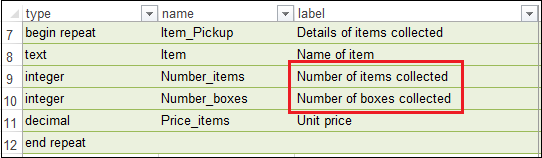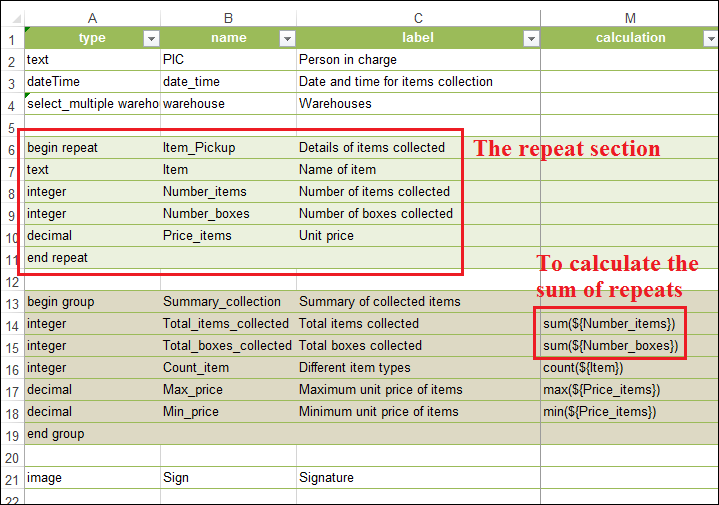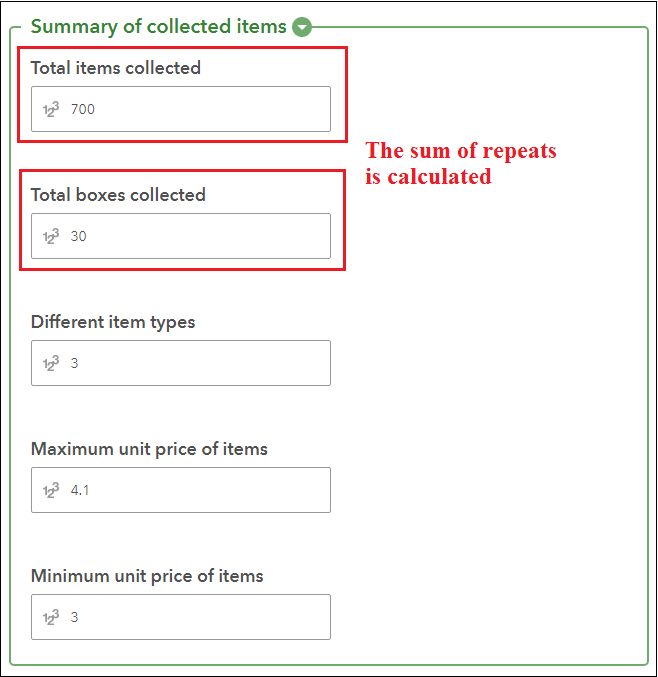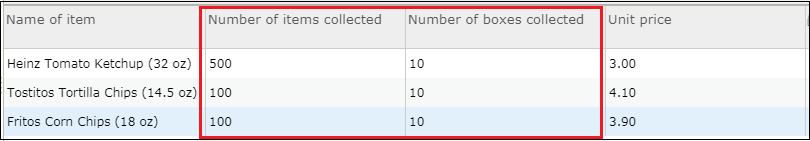English
Continue in the app
Be The First To Get Support Updates

# How To: Calculate the sum of repeats in ArcGIS Survey123

## Summary

Calculating the sum of repeats enables users to summarize the total value across multiple repeat questions answered in the same primary record. For example, to record the total items inspected during an asset inspection, to determine the total volume of chemical waste disposed by a chemical facility, or to identify the total tonnage of recyclable material collected from a residential area.

In this article, the repeat section comprises details of items collected from a few warehouses. The details of one item type is filled in one repeat record. The sum() function is used to calculate the total number of items and the total number of boxes collected from multiple repeat records based on the item types collected.## Procedure

The sum of repeats is calculated using the sum() function outside the repeat section. Follow the steps below to calculate the sum of repeats in ArcGIS Survey123.

1. Open ArcGIS Survey123 Connect and create a survey using an Advanced template.
2. Click the Open XLSForm spreadsheet icon to open the Excel form.
3. Configure the form to contain a repeat section.
4. Outside the repeat section, create an integer or decimal type question. In the calculation column, use the sum() function to calculate the sum of repeats.

The image below shows the sum() function configured in the calculation column outside the repeat section. The sum() function is used to calculate the total number of items and the total number of boxes collected based on the details provided in the repeat records.```Note:
Other aggregate functions such as count(), max(), and min() can also be calculated from the repeat records. These functions work with repeats within the same primary record, and not across repeat records in the survey.```
1. Save the Excel form and publish the survey. The image below shows the sum of all repeats calculated automatically in the survey form.When viewing the hosted feature layer in Map Viewer, the attribute table shows the sum of repeats and other aggregate functions configured in the survey form. The image below shows the sum of items and boxes collected in a single survey response with three repeat records.The individual records of each repeat record are stored in the related record table. The image below shows the details of the three repeat records sent in the single survey response. The Total items collected column in the attribute table is calculated based on the sum of the Number of items collected column in this related record table.## Related Information

Last Published: 4/27/2022

Article ID: 000021664

Software: Other Apps ArcGIS.Survey123.Connect ArcGIS Online Current